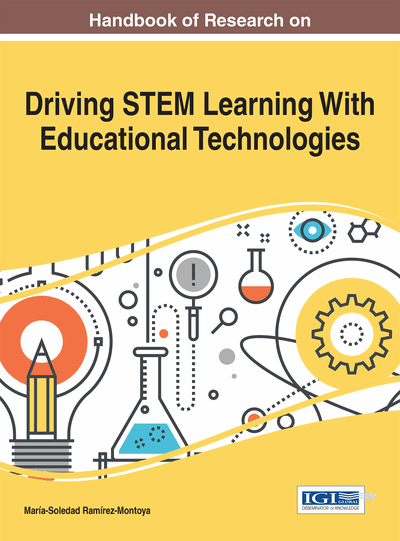# Teachers and Mathematical Modeling: What Are the Challenges?

Samantha Analuz Quiroz Rivera (Tecnologico de Monterrey, Mexico) and Ruth Rodríguez Gallegos (Tecnologico de Monterrey, Mexico)
Copyright: © 2017 |Pages: 20
DOI: 10.4018/978-1-5225-2026-9.ch003
OnDemand PDF Download:
Available
\$37.50
No Current Special Offers

## Abstract

Mathematics cannot be reduced to the use of algorithms. The main objective of teaching mathematics in school is their application in real world situations. Mathematical modeling was born as an answer of this concerned and implies the relation between mathematics and applications. Because of that, teachers need to be correctly training in the use of mathematical modeling in their daily lesson plans. The aim of this study is to propose a methodology for help teachers purchase mathematical modeling as a strategy to teach mathematics. Our methodology is based on the analysis of teachers' conceptions about learning and teaching mathematics and after that promote their evolution. The main characteristic is the collaborative work between teachers and the researcher in cycles of discussion and classes' implementation. The evidence showed that teachers can actually change their conceptions about what is needed for teaching mathematics and design lesson plans using mathematical modeling.
Chapter Preview
Top

## Introduction

One of the purposes of education is the development of mathematical literacy in students. Since it is during elementary school when basic notions of mathematics are introduced, this educational period needs to be taken into consideration in order to achieve comprehension of advanced mathematics required in universities. The Organization for Economic Co-operation and Development (OECD) define mathematical literacy as the use of mathematics in a variety of extra-mathematical contexts. According to that idea, mathematics methods and mechanical algorithms without context ought to not be promoted by educational institutions (OECD, 2010).

In Mexico, the Educational Reform on the basic educational level implemented in 2009 recognized that teaching of mathematics must follow the principles above mentioned. The Minister of Education described mathematics as a tool that will help students to solve real-world problems. Even more, one of the purposes of mathematics classes, in Mexico, is the development of mathematical modeling competencies. Mathematical modeling is defined as a didactical strategy that promotes the applications of mathematics (SEP, 2011).

Our research is focused on a phenomenon related to the above mentioned changes to the curriculum. Despite all the information given by the Minister of Education, mathematics is studied as a set of algorithms without any relation to real context. One of the main causes of that phenomenon is the huge distance from the curricula of elementary school and that of pre-service teachers. In Mexico, pre-service teachers’ curricula do not cover the learning of mathematical modeling (SEP, 2011). Beyond the use of the concept “mathematical modeling”, pre-service teachers have very few opportunities to reflect on the way they present mathematics to their students. The questions that emerge from this situation are: Why did the Reform of Education leave the teachers out of the equation? Is it possible for the teacher to implement a didactic strategy if he/she does not have the necessary training? How can pre-service teachers learn about mathematical modeling?

The present study, based on a doctoral dissertation, presents a training proposal implemented with pre-service teachers in Mexico. We consider a change is necessary in the curricula of the pre-service teachers’ universities. Nevertheless, it is not enough to memorize the concept of “mathematical modeling”, because that will not guarantee their correct application in the classroom. It is necessary to change the pre-service teachers’ conceptions of mathematical modeling.

In the following sections, we present, as a result of previous publications, six initial conceptions about mathematical modeling from pre-service teachers. Based on that research we described results of the present research question: How can the mathematical modeling conceptions of pre-service teachers evolve?

We proposed a methodology that combines a dialog between teachers and researcher in order to introduce the different elements of mathematical modeling through the constant guidance of the researcher for their application. The main objectives of the research are:

• To propose a methodology based on a theoretical knowledge that helps teachers evolve their conceptions regarding mathematical modeling.

• To describe the process of evolution of the conceptions and to identify the main elements that promoted that.

## Key Terms in this Chapter

Lesson Study: Methodology where teachers design and redesign lesson planning in a collaborative work.

Mathematical Modeling: Cycle that relates real world problems with mathematics models used or created by students.

Evolution of Conceptions: The change of conceptions generated by an epistemological obstacle.

Teaching Mathematical Modeling Cycle: Cycle of collaboration between teachers and researcher that promotes the evolving of conceptions.

Conceptions: Beliefs, attitudes, and consideration about certain topic. According with the Theory of the Conceptual Fields they are conformed by a situation, a set of schemas, symbolic representations and a control structure.

Applications: Real world situation where mathematical content is used.

## Complete Chapter List

Search this Book:
Reset